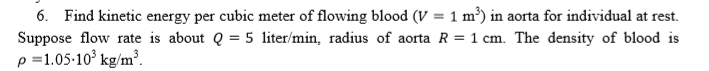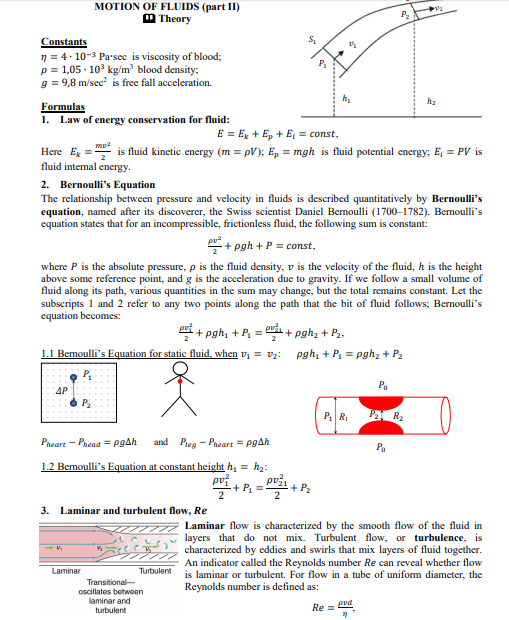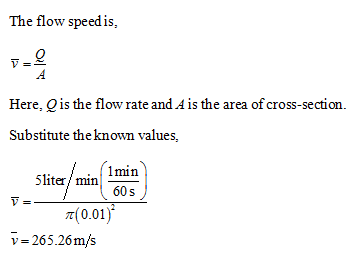# 6. Find kinetic energy per cubic meter of flowing blood (V = 1 m³) in aorta for individual at rest.Suppose flow rate is about Q = 5 liter/min, radius of aorta R = 1 cm. The density of blood isp =1.05-10° kg/m³. MOTION OF FLUIDS (part II)M TheoryConstantsn = 4- 10-3 Parsec is viscosity of blood;p= 1,05 - 10° kg/m blood density;g = 9,8 m/sec is free fall acceleration.hzFormulas1. Law of energy conservation for fluid:E = Ex + Ep + E, = const,Here Egfluid intemal energy.is fluid kinetic energy (m = pV); E, = mgh is fluid potential energy; E, = PV is2. Bernoulli's EquationThe relationship between pressure and velocity in fluids is described quantitatively by Bernoulli'sequation, named after its discoverer, the Swiss scientist Daniel Bemoulli (1700–1782). Bemoulli'sequation states that for an incompressible, frictionless fluid, the following sum is constant:+ pgh + P = const,where P is the absolute pressure, p is the fluid density, v is the velocity of the fluid, h is the heightabove some reference point, and g is the acceleration due to gravity. If we follow a small volume offluid along its path, various quantities in the sum may change, but the total remains constant. Let thesubscripts 1 and 2 refer to any two points along the path that the bit of fluid follows; Bernoulli'sequation becomes:Pri + pgh, + P, = i+ pghz + P..1.1 Bemoulli's Equation for static fluid, when v, = v2: pgh, + P, = pghz + P2PoAPPA R2P RPieg - Pneart = PgAhPheart - Phead = pgAhandPa1.2 Bemoulli's Equation at constant height h, = h2:pvž.-+ P =-+ P23. Laminar and turbulent flow, ReLaminar flow is characterized by the smooth flow of the fluid inlayers that do not mix. Turbulent flow, or turbulence, ischaracterized by eddies and swirls that mix layers of fluid together.An indicator called the Reynolds number Re can reveal whether flowis laminar or turbulent. For flow in a tube of uniform diameter, theReynolds number is defined as:LaminarTurbulentTransitional-oscillates betweenlaminar andturbulentpvdRe =

Question
4 viewshelp_outlineImage Transcriptionclose6. Find kinetic energy per cubic meter of flowing blood (V = 1 m³) in aorta for individual at rest. Suppose flow rate is about Q = 5 liter/min, radius of aorta R = 1 cm. The density of blood is p =1.05-10° kg/m³. fullscreenhelp_outlineImage TranscriptioncloseMOTION OF FLUIDS (part II) M Theory Constants n = 4- 10-3 Parsec is viscosity of blood; p= 1,05 - 10° kg/m blood density; g = 9,8 m/sec is free fall acceleration. hz Formulas 1. Law of energy conservation for fluid: E = Ex + Ep + E, = const, Here Eg fluid intemal energy. is fluid kinetic energy (m = pV); E, = mgh is fluid potential energy; E, = PV is 2. Bernoulli's Equation The relationship between pressure and velocity in fluids is described quantitatively by Bernoulli's equation, named after its discoverer, the Swiss scientist Daniel Bemoulli (1700–1782). Bemoulli's equation states that for an incompressible, frictionless fluid, the following sum is constant: + pgh + P = const, where P is the absolute pressure, p is the fluid density, v is the velocity of the fluid, h is the height above some reference point, and g is the acceleration due to gravity. If we follow a small volume of fluid along its path, various quantities in the sum may change, but the total remains constant. Let the subscripts 1 and 2 refer to any two points along the path that the bit of fluid follows; Bernoulli's equation becomes: Pri + pgh, + P, = i+ pghz + P.. 1.1 Bemoulli's Equation for static fluid, when v, = v2: pgh, + P, = pghz + P2 Po AP PA R2 P R Pieg - Pneart = PgAh Pheart - Phead = pgAh and Pa 1.2 Bemoulli's Equation at constant height h, = h2: pvž. -+ P =- + P2 3. Laminar and turbulent flow, Re Laminar flow is characterized by the smooth flow of the fluid in layers that do not mix. Turbulent flow, or turbulence, is characterized by eddies and swirls that mix layers of fluid together. An indicator called the Reynolds number Re can reveal whether flow is laminar or turbulent. For flow in a tube of uniform diameter, the Reynolds number is defined as: Laminar Turbulent Transitional- oscillates between laminar and turbulent pvd Re = fullscreen
check_circle

Step 1...

### Want to see the full answer?

See Solution

#### Want to see this answer and more?

Solutions are written by subject experts who are available 24/7. Questions are typically answered within 1 hour.*

See Solution
*Response times may vary by subject and question.
Tagged in